# MP Board Class 6th Maths Solutions Chapter 14 Practical Geometry Ex 14.2

## MP Board Class 6th Maths Solutions Chapter 14 Practical Geometry Ex 14.2

Question 1.
Draw a line segment of length 7.3 cm using a ruler.
Solution:
Steps of construction :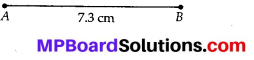(i) Place the zero mark of the ruler at a point A.
(ii) Mark a point B at a distance of 7.3 cm from A.
(iii) Join AB.
$$\overline{A B}$$ is the required line segment of length 7.3 cm.

Question 2.
Construct a line segment of length 5.6 cm using ruler and compasses.
Solution:
Steps of construction :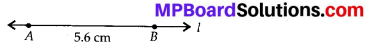(i) Draw a line l. Mark a point A on this line.
(ii) Place the compasses pointer on zero mark of the ruler. Open it to place the pencil point upto 5.6 cm mark.
(iii) Without changing the opening of the compasses, place the pointer on A and draw an arc that cuts l at B.
$$\overline{A B}$$ is the required line segment of length 5.6 cm.

Question 3.
Construct $$\overline{A B}$$ of length 7.8 cm. From this, cut off $$\overline{A C}$$ of length 4.7 cm. Measure $$\overline{B C}$$.
Solution:
Steps of construction :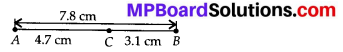(i) Place the zero mark of the ruler at A.
(ii) Mark a point B at a distance 7.8 cm from A.
(iii) Again, mark a point C at a distance 4.7 from A.
(iv) By measuring $$\overline{B C}$$, we find that BC = 3.1 cm.Question 4.
Given $$\overline{A B}$$ of length 3.9 cm, construct $$\overline{P Q}$$ such that the length of $$\overline{P Q}$$ is twice that of $$\overline{A B}$$. Verify by measurement.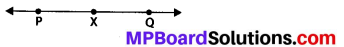(Hint: Construct $$\overline{P X}$$ such that length of $$\overline{P X}$$ = length of $$\overline{A B}$$; then cut off $$\overline{X Q}$$ such that $$\overline{X Q}$$ also has the length of $$\overline{A B}$$.)
Solution: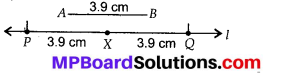Steps of construction :
(i) Draw a line ‘l’.
(ii) Construct $$\overline{P X}$$ such that length of $$\overline{P X}$$ = length of $$\overline{A B}$$ = 3.9 cm
(iii) Then cut of $$\overline{X Q}$$ such that $$\overline{X Q}$$ also has the length of $$\overline{A B}$$.
(iv) Thus the length of $$\overline{P X}$$ and the length of $$\overline{X Q}$$ added together make twice the length of $$\overline{A B}$$.
Verification:
By measurement we find that
PQ = 7.8 cm = 3.9 cm + 3.9 cm
= $$\overline{A B}$$ + $$\overline{A B}$$ = 2 × $$\overline{A B}$$Question 5.
Given $$\overline{A B}$$ of length 7.3 cm and $$\overline{C D}$$ of length 3.4 cm, construct a line segment $$\overline{X Y}$$ such that the length of $$\overline{X Y}$$ is equal to the difference between the lengths of $$\overline{A B}$$ and $$\overline{C D}$$. Verify by measurement.
Solution:
Steps of construction :
(i) Draw a line ‘l’ and take a point X on it.
(ii) Construct $$\overline{X Z}$$ such that length $$\overline{X Z}$$ = length of $$\overline{A B}$$ = 7.3 cm.
(iii) Then cut off $$\overline{Z Y}$$ = length of $$\overline{C D}$$ = 3.4 cm
(iv) Thus the length of $$\overline{X Y}$$ = length of $$\overline{A B}$$ – length of $$\overline{C D}$$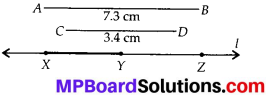Verification:
By measurement, we find that length of $$\overline{X Y}$$ = 3.9 cm
= 7.3 cm – 3.4 cm = $$\overline{A B}-\overline{C D}$$